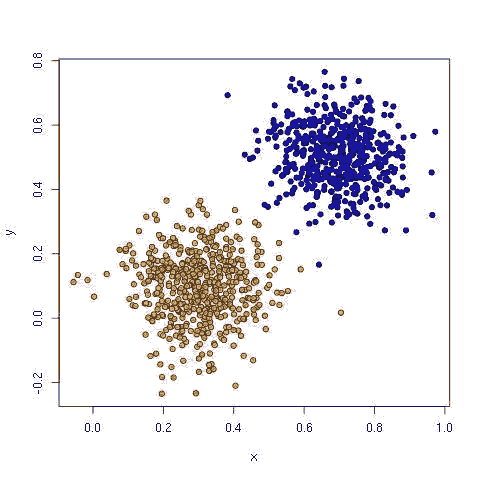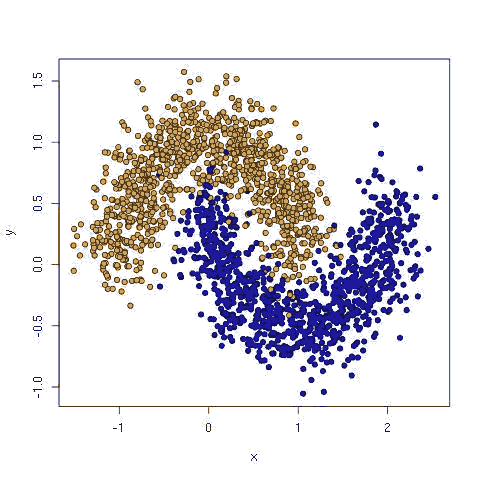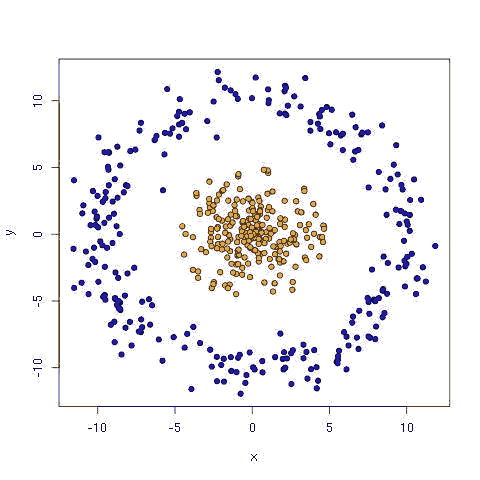# Tensorflow简单教程

### 为什么使用Tensorflow?

作为一个数据科学研究者，已经有很多工具如R语言、Scikit等学习工具，为什么还要使用Tensorflow呢？

1. TensorFlow的深度学习部分能够在一个模型中堆积了许多不同的模型和转换，你能够在一个模型中方便地处理文本 图片和规则分类以及连续变量，同时实现多目标和多损失工作；

2. TensorFlow的管道部分能够将数据处理和机器学习放在一个框架中，TensorFlow指引了方向。

### Titanic 数据集的简单模型

开始一个简单的案例，从Kaggle获得Titanic 数据集，首先，肯定你已经安装了 TensorFlow 和 Scikit Learn，包括一些支持库包Scikit Flow ，它可以简化TensorFlow的许多工作。

`pip install numpy scipy sklearn pandas# For Ubuntu:pip install https://storage.googleapis.com/tensorflow/linux/cpu/tensorflow-0.5.0-cp27-none-linux_x86_64.whl# For Mac:pip install https://storage.googleapis.com/tensorflow/mac/tensorflow-0.5.0-py2-none-any.whlpip install git+git://github.com/google/skflow.git`

`git clone https://github.com/ilblackdragon/tf_examples.git`

`>>> import pandas>>> data = pandas.read_csv('data/train.csv')>>> data.shape(891, 12)>>> data.columnsIndex([u'PassengerId', u'Survived', u'Pclass', u'Name', u'Sex', u'Age',       u'SibSp', u'Parch', u'Ticket', u'Fare', u'Cabin', u'Embarked'],      dtype='object')>>> data[:1]   PassengerId  Survived  Pclass                     Name   Sex  Age  SibSp  0            1         0       3  Braund, Mr. Owen Harris  male   22      1   Parch     Ticket  Fare Cabin Embarked0      0  A/5 21171  7.25   NaN        S`

`>>> y, X = train['Survived'], train[['Age', 'SibSp', 'Fare']].fillna(0)>>> X_train, X_test, y_train, y_test = train_test_split(X, y, test_size=0.2, random_state=42)>>> lr = LogisticRegression()>>> lr.fit(X_train, y_train)>>> print accuracy_score(lr.predict(X_test), y_test)0.664804469274`

`>>> import skflow>>> import random>>> random.seed(42) # to sample data the same way>>> classifier = skflow.TensorFlowLinearClassifier(n_classes=2, batch_size=128, steps=500, learning_rate=0.05)>>> classifier.fit(X_train, y_train)>>> print accuracy_score(classifier.predict(X_test), y_test)0.68156424581`

### Scikit Flow

Scikit Flow是一个将TensorFlow包装其中提供多新与Scikit Learn API类似的API。TensorFlow是一个构建和执行图，这是一个强大的概念，但是开始时比较复杂一些。所以，需要简化包装一下。

• TensorFlowTrainer — 各种优化类，可以进行灰度修剪等优化。
• logistic_regression逻辑回归 — 创建一个逻辑回归模型的图
• linear_regression线性回归 — 创建线性回归模型的图。
• DataFeeder — 取样训练数据的最小批次放入模型。
• TensorFlowLinearClassifier — 一个使用LogisticRegression实现Scikit Learn接口的类，它创建一个模型和一个训练者，使用给定数据集调用fit()运行训练者，调用predict()在评估模型中运行模型。
• TensorFlowLinearRegressor — 类似于TensorFlowClassifier, 但是使用 LinearRegression作为一个模型

### 模拟数据

我们使用TensorFlow分析三种模拟数据：线性可数据，月亮和土星数据三种。第一种使用线性分类器很容易实现，后两种需要非线性模型如多层神经网络multi-layer neural network。Jason Baldridge在其github上根据Tensorflow案例mnist.py编写了Softmax回归： softmax.py.，使用它进行线性分析：

\$ python softmax.py --train simdata/linear_data_train.csv --test simdata/linear_data_eval.csv
Accuracy: 0.99

\$ python softmax.py --train simdata/linear_data_train.csv --test simdata/linear_data_eval.csv --num_epochs 2
Accuracy: 1.0

Hello, TensorFlow入门教程

TensorFlow案例源码